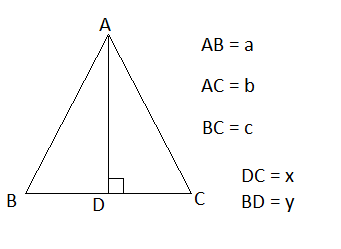# Defeating Heron

Let a ∆ABCNow area of the triangle =$$\frac{\sqrt{a^{2} × b^{2} - q^{2}}}{2}$$

Where q = $\frac{c^{2} - a^{2} - b^{2}}{2}$

Proof:

x + y = c

h = AD

x = $\sqrt{b^{2} - h^{2}}$

y = $\sqrt{a^{2} - h^{2}}$

c = $\sqrt{b^{2} - h^{2}}$ + $\sqrt{a^{2} - h^{2}}$

$c^{2}$ = $(\sqrt{b^{2} - h^{2}} + \sqrt{a^{2} - h^{2}})^{2}$

$c^{2}$ = $a^{2} + b^{2} - 2h^{2} + 2\sqrt{(a^{2} - h^{2})(b^{2} - h^{2})}$

$2\sqrt{(a^{2} - h^{2})(b^{2} - h^{2})}$ = $c^{2} - a^{2} - b^{2} +2h^{2}$

$(a^{2} - h^{2})(b^{2} - h^{2})$ = $(\frac{c^{2} - a^{2} - b^{2} }{2} + h^{2})^{2}$

Let q = $\frac{c^{2} - a^{2} -b^{2}}{2}$ and solve left side further and cancelling $h^{4}$ from both sides we get

$a^{2} × b^{2} - (a^{2} + b^{2})h^{2}$ = $q^{2} +2qh^{2}$

$a^{2} × b^{2} - q^{2}$ = $h^{2}(2q + a^{2} + b^{2})$ ...(1)

Now as $2q$ = $c^{2} - a^{2} - b^{2}$

Therefore $2q + a^{2} + b^{2}$ = $c^{2}$. Putting this into (1)

$h^{2} × c^{2}$ = $a^{2} × b^{2} - q^{2}$

$h$ = $\frac{\sqrt{a^{2} × b^{2} - q^{2}}}{c}$

Now as area = $\frac{1}{2}ch$

Area = $\frac{\sqrt{a^{2} × b^{2} - q^{2}}}{2}$

Hence proved

Try this new formula and check if you find it usefulNote by Sahar Bano
1 year, 2 months ago

This discussion board is a place to discuss our Daily Challenges and the math and science related to those challenges. Explanations are more than just a solution — they should explain the steps and thinking strategies that you used to obtain the solution. Comments should further the discussion of math and science.

When posting on Brilliant:

• Use the emojis to react to an explanation, whether you're congratulating a job well done , or just really confused .
• Ask specific questions about the challenge or the steps in somebody's explanation. Well-posed questions can add a lot to the discussion, but posting "I don't understand!" doesn't help anyone.
• Try to contribute something new to the discussion, whether it is an extension, generalization or other idea related to the challenge.
• Stay on topic — we're all here to learn more about math and science, not to hear about your favorite get-rich-quick scheme or current world events.

MarkdownAppears as
*italics* or _italics_ italics
**bold** or __bold__ bold
- bulleted- list
• bulleted
• list
1. numbered2. list
1. numbered
2. list
Note: you must add a full line of space before and after lists for them to show up correctly
paragraph 1paragraph 2

paragraph 1

paragraph 2

[example link](https://brilliant.org)example link
> This is a quote
This is a quote
    # I indented these lines
# 4 spaces, and now they show
# up as a code block.

print "hello world"
# I indented these lines
# 4 spaces, and now they show
# up as a code block.

print "hello world"
MathAppears as
Remember to wrap math in $$ ... $$ or $ ... $ to ensure proper formatting.
2 \times 3 $2 \times 3$
2^{34} $2^{34}$
a_{i-1} $a_{i-1}$
\frac{2}{3} $\frac{2}{3}$
\sqrt{2} $\sqrt{2}$
\sum_{i=1}^3 $\sum_{i=1}^3$
\sin \theta $\sin \theta$
\boxed{123} $\boxed{123}$

## Comments

Sort by:

Top Newest

Where did you find this, @Sahar Bano? This is really good!

- 1 year, 1 month ago

Log in to reply

Just figured it out myself

- 1 year, 1 month ago

Log in to reply

Nice!, @Sahar Bano

- 1 year, 1 month ago

Log in to reply

×

Problem Loading...

Note Loading...

Set Loading...